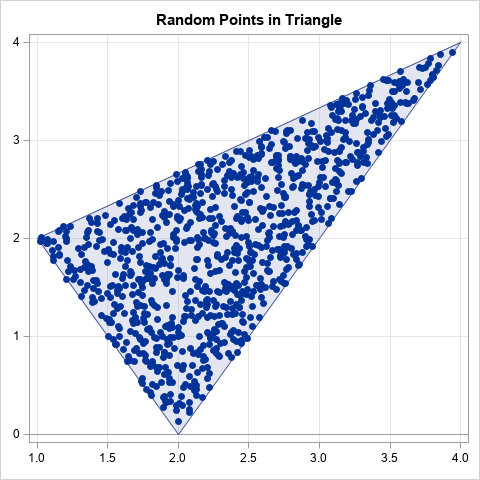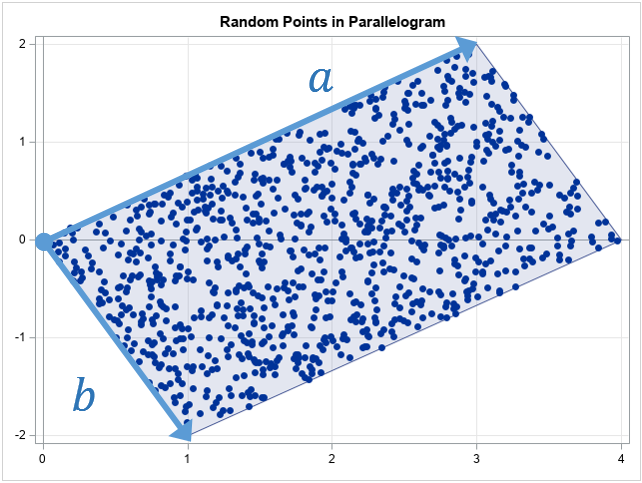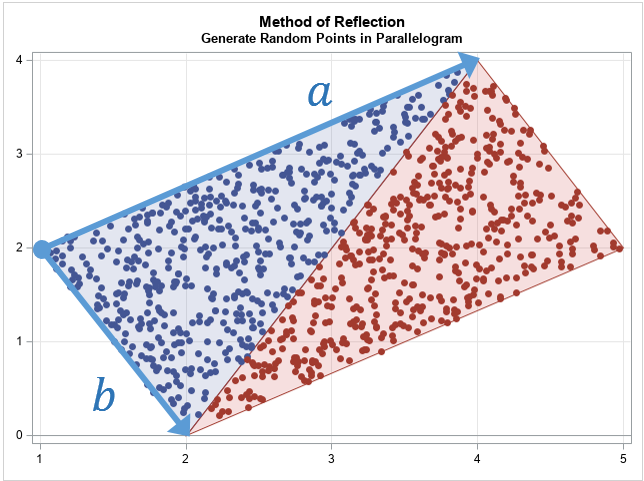How can you efficiently generate N random uniform points in a triangular region of the plane? There is a very cool algorithm (which I call the reflection method) that makes the process easy. I no longer remember where I saw this algorithm, but it is different from the "weighted average" method in Devroye (1986, p. 569-570). This article describes and implements the reflection algorithm for generating random points in a triangle from the uniform distribution. The graph to the right shows 1,000 random points in the triangle with vertices P1=(1, 2), P2=(4, 4), and P3=(2, 0). The method works for any kind of triangle: acute, obtuse, equilateral, and so forth.

In this article, "random points" means that the points are drawn randomly from the uniform distribution.

### Random points in a parallelogram

The easiest way to understand the algorithm is to think about generating points in a parallelogram. For simplicity, translate the parallelogram so that one vertex is at the origin. Two sides of the parallelogram share that vertex. Let a and b be the vectors from the origin to the adjacent vertices.

To produce a random point in the parallelogram, generate u1, u2 ~ U(0,1) and form the vector sum
p = u1*a + u2*b
This is the 2-D parameterization of the parallelogram, so for random u1 and u2, the point p is uniformly distributed in the parallelogram. The geometry is shown in the following figure.The following SAS/IML program generates 1,000 random points in the parallelogram. The graph is shown above.

```proc iml; n = 1000; call randseed(1234);   /* random points in a parallelgram */ a = {3 2}; /* vector along one side */ b = {1 -2}; /* vector along adjacent side */ u = randfun(n // 2, "Uniform"); /* u[,1], u[,2] ~ U(0,1) */ w = u[,1]@a + u[,2]@b; /* linear combination of a and b */ title "Random Points in Parallelogram"; call scatter(w[,1], w[,2]) grid={x,y};```

The only mysterious part of the program is the use of the Kronecker product (the '@' operator) to form linear combinations of the a and b vectors. The details of the Kronecker product operator are described in a separate article. Briefly, it is an efficient way to generate linear combinations of a and b without writing a loop.

### The reflection step

A useful fact about random uniform variates is that if u ~ U(0,1), then also v = 1 - u ~ U(0,1). You can use this fact to convert N points in a parallelogram into N points in a triangle.

Let u1, u2 ~ U(0,1) be random variates in (0,1). If u1 + u2 ≤ 1, then the vector u1*a + u2*b is in the triangle with sides a and b. If u1 + u2 > 1, then define v1 = 1 - u1 and v2 = 1 - u2, which are also random uniform variates. You can verify that the sum v1 + v2 ≤ 1, which means that the vector v1*a + v2*b is in the triangle with sides a and b.

This is shown in the following graph. The blue points are the points for which u1 + u2 ≤ 1. The red points are for u1 + u2 > 1. When you form v1 and v2, the red triangle get reflected twice and ends up on top of the blue triangle. The two reflections are equivalent to a 180 degree rotation about the center of the parallelogram, which might be easier to visualize.### Generate random points in a triangle

With this background, you can now generate random points in any triangle. Let P1, P2, and P3 be the vertices of the triangle. The algorithm to generate random points in the triangle is as follows:

1. Define the vectors a = P2 - P1 and b = P3 - P1. The vectors define the sides of the triangle when it is translated to the origin.
2. Generate random uniform values u1, u2 ~ U(0,1)
3. If u1 + u2 > 1, apply the transformation u1 → 1 - u1 and u2 → 1 - u2.
4. Form w = u1 a + u2 b, which is a random point in the triangle at the origin.
5. The point w + P1 is a random point in the original triangle.

The following SAS/IML program implements this algorithm and runs it for the triangle with vertices P1=(1, 2), P2=(4, 4), and P3=(2, 0).

```/* generate random uniform sample in triangle with vertices P1 = (x0,y0), P2 = (x1,y1), and P3 = (x2,y2) The triangle is specified as a 3x2 matrix, where each row is a vertex. */ start randUnifTriangle(P, n); a = P[2,] - P[1,]; /* translate triangle to origin */ b = P[3,] - P[1,]; /* a and b are vectors at the origin */ u = randfun(n // 2, "Uniform"); idx = loc(u[,+] >= 1); /* identify points outside of the triangle */ if ncol(idx)>0 then u[idx,] = 1 - u[idx,]; /* transform variates into the triangle */ w = u[,1]@a + u[,2]@b; /* linear combination of a and b vectors */ return( P[1,] + w ); /* translate triangle back to original position */ finish; store module=(randUnifTriangle);   /* triangle contains three vertices */ call randseed(1234,1); P = {1 2, /* P1 */ 4 4, /* P2 */ 2 0}; /* P3 */ n = 1000; w = randUnifTriangle(P, n);   title "Random Points in Triangle"; ods graphics / width=480px height=480px; call scatter(w[,1], w[,2]) grid={x,y};```

The graph of the 1,000 random points appears at the top of this program.

### Showing scatter plots with polygons

As written, the programs in this article create scatter plots that show the random points. To improve the exposition, I used the polygon package to draw graphs that overlay the scatter plot and a polygon. You can download and install the polygon package if you have PROC IML with SAS 9.4m3 or later. You can download the complete SAS program that performs all the computations and creates all the graphs in this article.

### Summary

In summary, this article shows how to generate random uniform points in a triangle by using the reflection algorithm. The reflection algorithm is based on generating random points in a parallelogram. If you draw the diagonal of a parallelogram, you get two congruent triangles. The algorithm reflects (twice) all points in one triangle into the other triangle. The algorithm is implemented in SAS by using the SAS/IML language, although you could also use the SAS DATA step.

ShareDistinguished Researcher in Computational Statistics

Rick Wicklin, PhD, is a distinguished researcher in computational statistics at SAS and is a principal developer of SAS/IML software. His areas of expertise include computational statistics, simulation, statistical graphics, and modern methods in statistical data analysis. Rick is author of the books Statistical Programming with SAS/IML Software and Simulating Data with SAS.

1.Rick,
That's spiffing method to generate random points in triangular/parallelogram region of the plane!
Could you show how to generate random point in a given center of circle?

Thank you.
Ethan

2.Isn't there a mistake in here:
If u1 + u2 > 1, then define v1 = 1 - u1 and v2 = 1 - u2, which are also random uniform variates. You can verify that the sum v1 + v2 ≤ 1, which means that the vector v1*a + v2*b is in the triangle with sides a and b.
So if u1=.55, u2=.55, then u1+u2>1, then v1=-.45, v2=-.45 which makes a vector v1*a + v2*b outside the parallelogram no?

•No mistake. 1 - 0.55 = 0.45, not -0.45. Thus, v1 = v2 = 0.45 and v1*a + v2*b is inside the triangle.